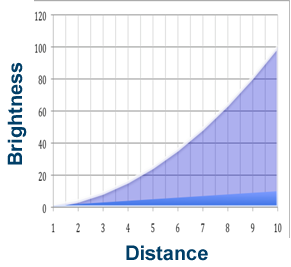# 1920 Activity: Inverse Square LawThe principle of the inverse square law is best illustrated with a simple diagram.  As light from a source spreads out in all directions, it gets fainter with distance.  The three disks shown at right are at one, two, and three times the distance from a star.  Since the area of each disk increases with the square of its diameter, the concentration of light on each part of the disk must decrease in proportion to the square of the distance to the light source.This chart shows the inverse square relationship for a larger range of distances.  For example, imagine two Cepheid variables that have the same period, and therefore the same brightness.  Star A is nearby and known to be 10 light years distant.  Star B is in a globular cluster and is 40 times fainter.  The chart shows that a difference of 40 times in brightness corresponds to a difference of 6.3 in distance.  So the globular cluster must be 63 light years away.

Use the chart or calculate to figure out the following distances, then place your mouse over the Answer button to see if your answer agrees with the experts.

Star A is 10 light years away, and Star B is 36 times fainter.

Star A is 10 light years away, and Star B is 81 times fainter.

Star A is 5 light years away, and Star B is 81 times fainter.